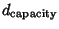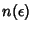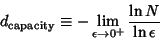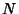## Capacity Dimension

A Dimension also called the Fractal Dimension, Hausdorff Dimension, and Hausdorff-Besicovitch Dimension in which nonintegral values are permitted. Objects whose capacity dimension is different from their Topological Dimension are called Fractals. The capacity dimension of a compact Metric Spaceis a Real Numbersuch that ifdenotes the minimum number of open sets of diameter less than or equal to, thenis proportional toas. Explicitly,(if the limit exists), whereis the number of elements forming a finite Cover of the relevant Metric Space andis a bound on the diameter of the sets involved (informally,is the size of each element used to cover the set, which is taken to approach 0). If each element of a Fractal is equally likely to be visited, then, whereis the Information Dimension. The capacity dimension satisfieswhereis the Correlation Dimension, and is conjectured to be equal to the Lyapunov Dimension.

References

Nayfeh, A. H. and Balachandran, B. Applied Nonlinear Dynamics: Analytical, Computational, and Experimental Methods. New York: Wiley, pp. 538-541, 1995.

Peitgen, H.-O. and Richter, D. H. The Beauty of Fractals: Images of Complex Dynamical Systems. New York: Springer-Verlag, 1986.

Wheeden, R. L. and Zygmund, A. Measure and Integral: An Introduction to Real Analysis. New York: M. Dekker, 1977.